Pull Down & Release to Refresh

# Linear Equation Revision Exercises

Would you like to test yourself to see how much you know about linear equations? Have a go at the exercises in this article. When you're finished, check out the solutions page to see how well you did.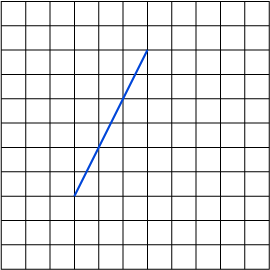1. Find the gradient (slope) of this line segment.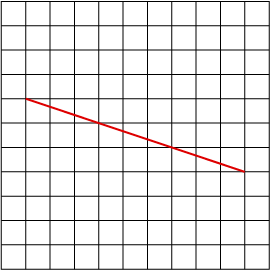2. Find the gradient (slope) of this line segment.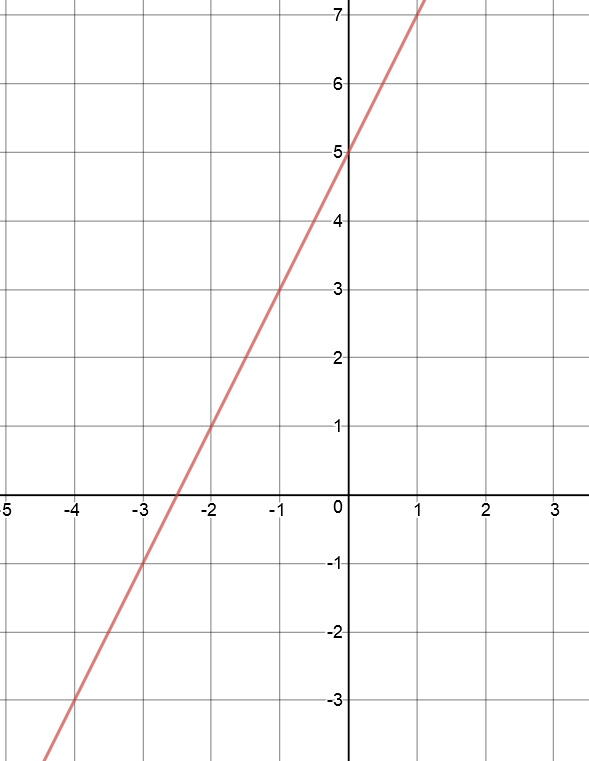3. What is the $y$-intercept of this line?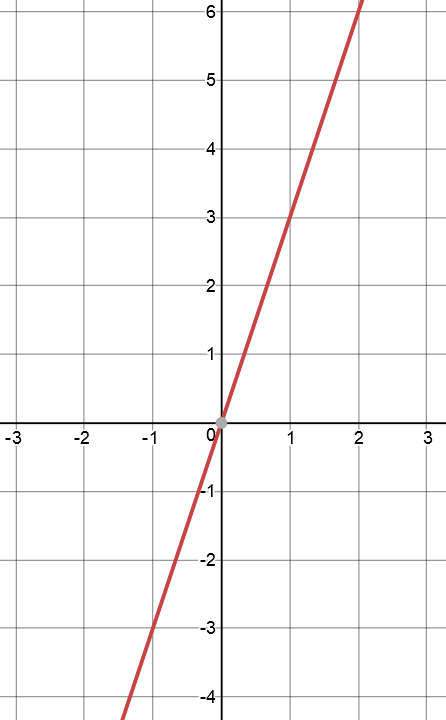4. What is the equation of this line?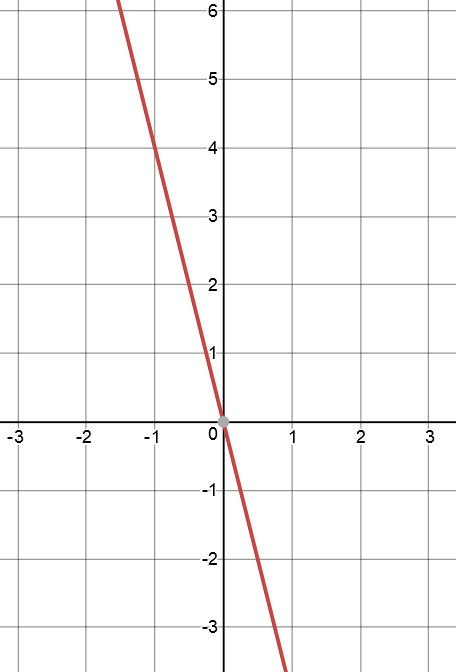5. What is the equation of this line?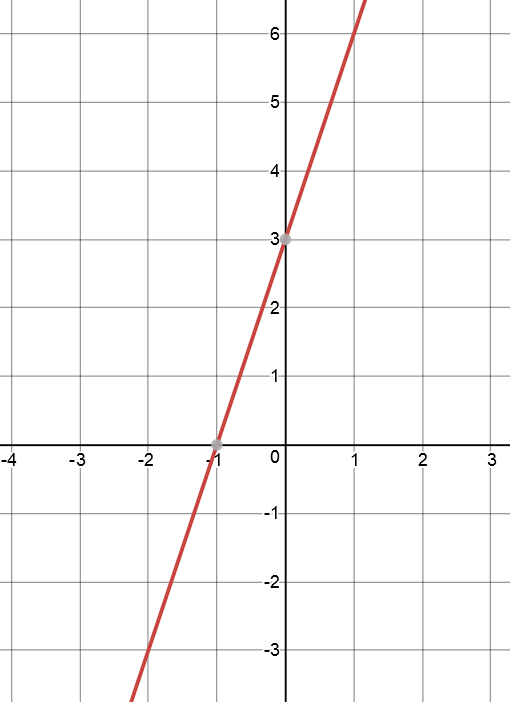6. What is the equation of this line?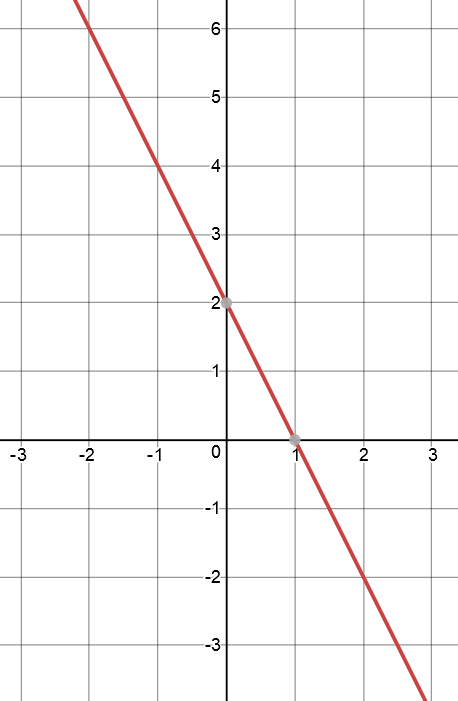7. What is the equation of this line?

8. Which of these lines has the equation $y = 2x + 3$?

A.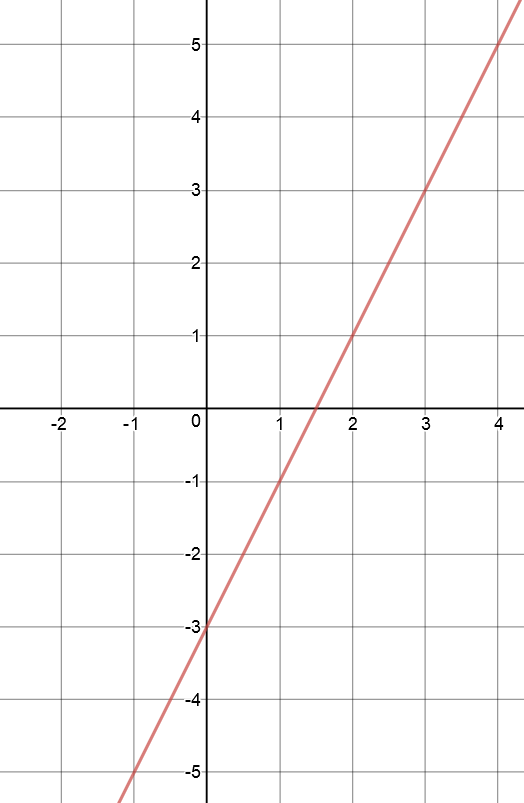B.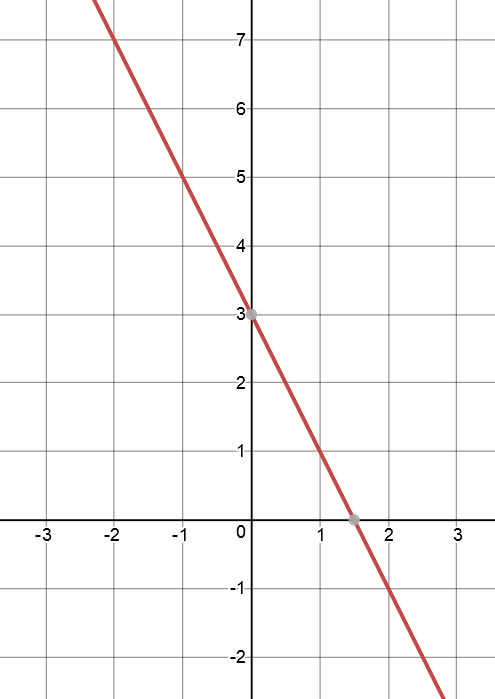C.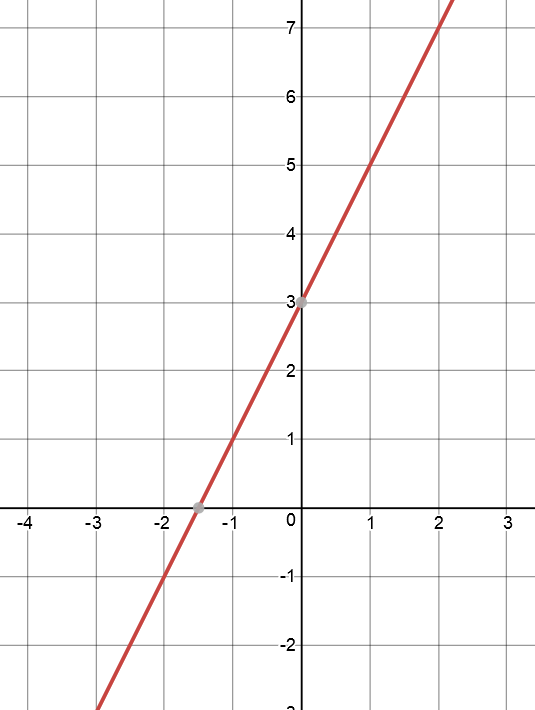9. Which of these lines has the equation $y = 2 - x$?

A.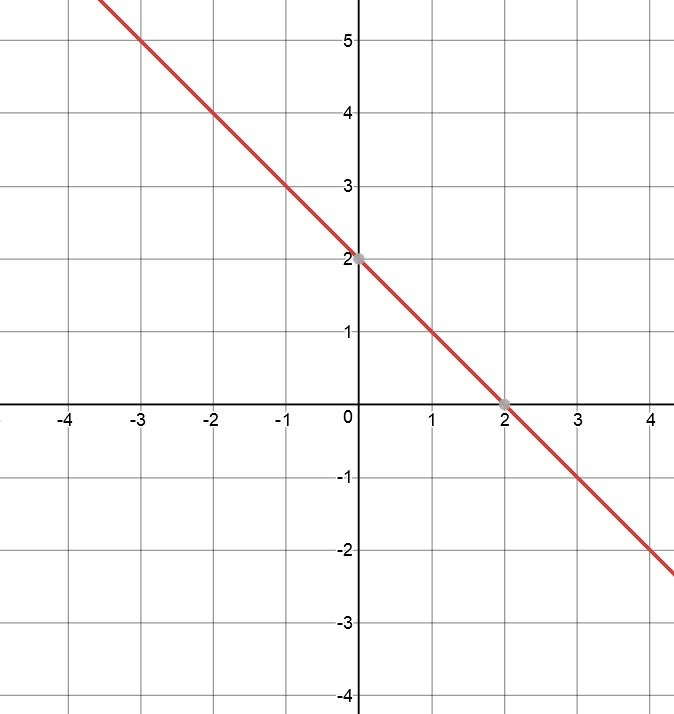B.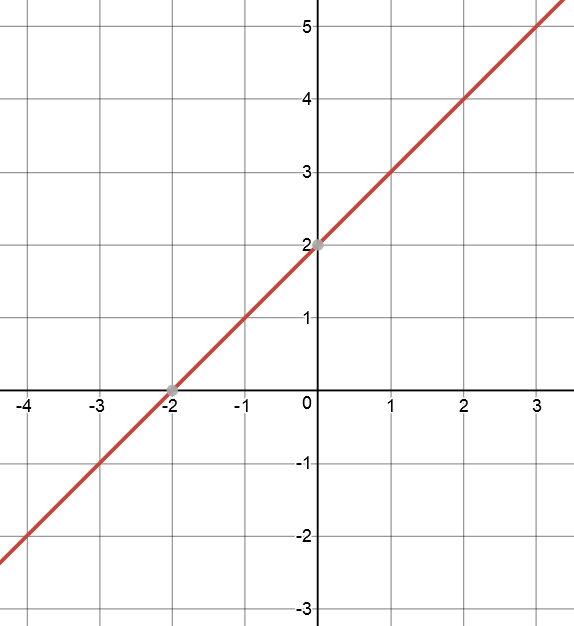C.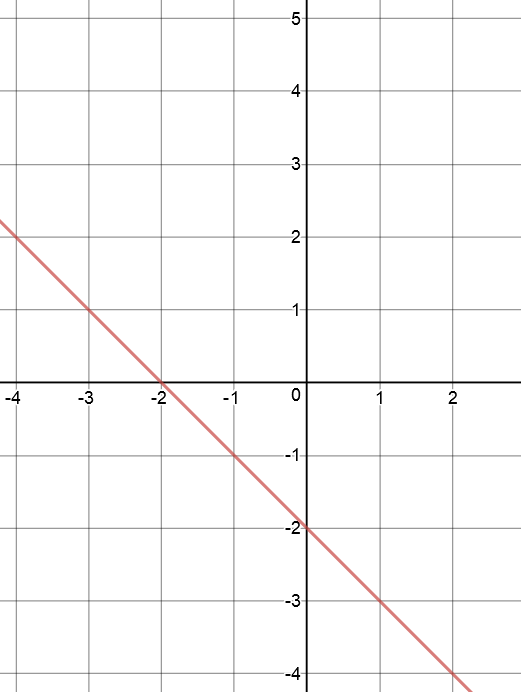All done? Now check out the solutions page to see how you did.

### Description

coordinate geometry is a branch of geometry where the position of the points on the plane is defined with the help of an ordered pair of numbers also known as coordinates. In this tutorial series, you will learn about vast range of topics such as Cartesian Coordinates, Midpoint of a Line Segment etc

### Audience

year 10 or higher, several chapters suitable for Year 8+ students.

### Learning Objectives

Explore topics related to Coordinates Geometry

Author: Subject Coach
You must be logged in as Student to ask a Question.